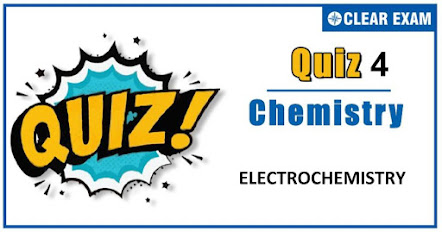## [LATEST]\$type=sticky\$show=home\$rm=0\$va=0\$count=4\$va=0

Q1. The resistance of a decinormal solution of a salt occupying a volume between two platinum electrodes 1.80 cm apart and 5.4 cm^2 in area was formed to be 32 ohm. The specific and equivalent conductivity respectively in their proper units are :
•  104.1 and 0.0104
•  None of these
•  208.2 and 0.0208
•  0.0104 and 104.0
Solution
k=1/R ×1/a=1/32 ×1.8/5.4 = 0.0104 And λ= k × V=0.0104 × 10,000=104

Q2.Standard reduction electrode potentials of three metals A,B and C are respectively + 0.5 V, - 3.0 V and – 1.2 V. The reducing powers of these metals are
•  A>B>C
•  B>C>A
•  A>C>B
•  C>B>A
Solution
More the reduction potential, more is the power to get itself reduced or lesser is reducing power or greater is oxidizing power

Q3.  When a quantity of electricity is passed through CuSO_4 solution, 0.16 g of copper gets deposited. If the same quantity of electricity is passed through acidulated water, then the volume of H_2 liberated at STP will be [Given , atomic weight of Cu = 64]
•   〖4.0 cm〗^3
•  〖56 cm〗^3
•  〖604 cm〗^3
•  〖8.0 cm〗^3
Solution

Q4. A student made the following observations in the laboratory, i) Clean copper metal did not react with 1 molar Pb(NO_3 )_2 solution. ii) Clean lead metal dissolved in a 1 molar AgNO_3 solution and crystals of Ag metal appeared. iii) Clean silver metal did not react with 1 molar Cu(NO_3 )_2 solution. The order of decreasing reducing character of the three metals is :
•  Cu,Pb,Ag
•  Cu,Ag,Pb
•  Pb,Ag,Cu
•  Pb,Cu,Ag
Solution
Metal placed above in electrochemical series replaces the other from its salt solutions.

Q5.Resistance of a conductivity cell filled with a solution of an electrolyte of concentration 0.1 M is 100Ω. The conductivity of this solution is 1.29 S m^(-1). Resistance of the same cell when filled with 0.2 M of the same solution is 520Ω. The molar conductivity of 0.02 M solution of the electrolyte will be
•  12.4×〖10〗^(-4) S m^2 mol^(-1)
•  1.24×〖10〗^(-4) S m^2 mol^(-1)
•  1240×〖10〗^(-4) S m^2 mol^(-1)
•  124×〖10〗^(-4) S m^2 mol^(-1)
Q6. In electrolysis, oxidation takes place at:
•  The surface of electrolyte solution
•  Anode
• Cathode
•  The anode as well as cathode
Solution
Anode is electrode at which oxidation occurs.

Q7.For which case Λ values vs√c show a straight line?
•  CH_3 COOH
•  CH_3 NH_2
•  KCl
•  HCOOH
Solution
For strong electrolytes Λ vs √c plots are straight line

Q8.Normal Al-AlCl_3 coupled with normal hydrogen electrode gives an e.m.f. of 1.66 V. The standard oxidation electrode potential of aluminium is :
•  + 0.83 V
•  + 1.66 V
•  - 1.66 V
•  - 0.83 V
Solution

Q9.The ionic conductivity of H^+ and OH^- at 298 K are 349.8 and 198.5 mho cm^2 eq ^(-1) respectively. The equivalent conductivity of H_2 O at infinite dilution is :
•  54.83
•  699.6
•  151.3
•  548.3
Solution#### Written by: AUTHORNAME

AUTHORDESCRIPTION## Want to know more

Please fill in the details below:

## Latest NEET Articles\$type=three\$c=3\$author=hide\$comment=hide\$rm=hide\$date=hide\$snippet=hide

Name

ltr
item
BEST NEET COACHING CENTER | BEST IIT JEE COACHING INSTITUTE | BEST NEET, IIT JEE COACHING INSTITUTE: ELECTROCHEMISTRY QUIZ-4
ELECTROCHEMISTRY QUIZ-4
https://1.bp.blogspot.com/-RLwewzo1h0I/X5u6MCE0hVI/AAAAAAAAGzw/HKD-4m4NV7sPD_emgR_PgvWuYTb55nvOgCLcBGAsYHQ/w444-h232/Quiz%2BImage%2BTemplate%2B%252823%2529.jpg
https://1.bp.blogspot.com/-RLwewzo1h0I/X5u6MCE0hVI/AAAAAAAAGzw/HKD-4m4NV7sPD_emgR_PgvWuYTb55nvOgCLcBGAsYHQ/s72-w444-c-h232/Quiz%2BImage%2BTemplate%2B%252823%2529.jpg
BEST NEET COACHING CENTER | BEST IIT JEE COACHING INSTITUTE | BEST NEET, IIT JEE COACHING INSTITUTE
https://www.cleariitmedical.com/2020/10/electrochemistry-quiz-4.html
https://www.cleariitmedical.com/
https://www.cleariitmedical.com/
https://www.cleariitmedical.com/2020/10/electrochemistry-quiz-4.html
true
7783647550433378923
UTF-8